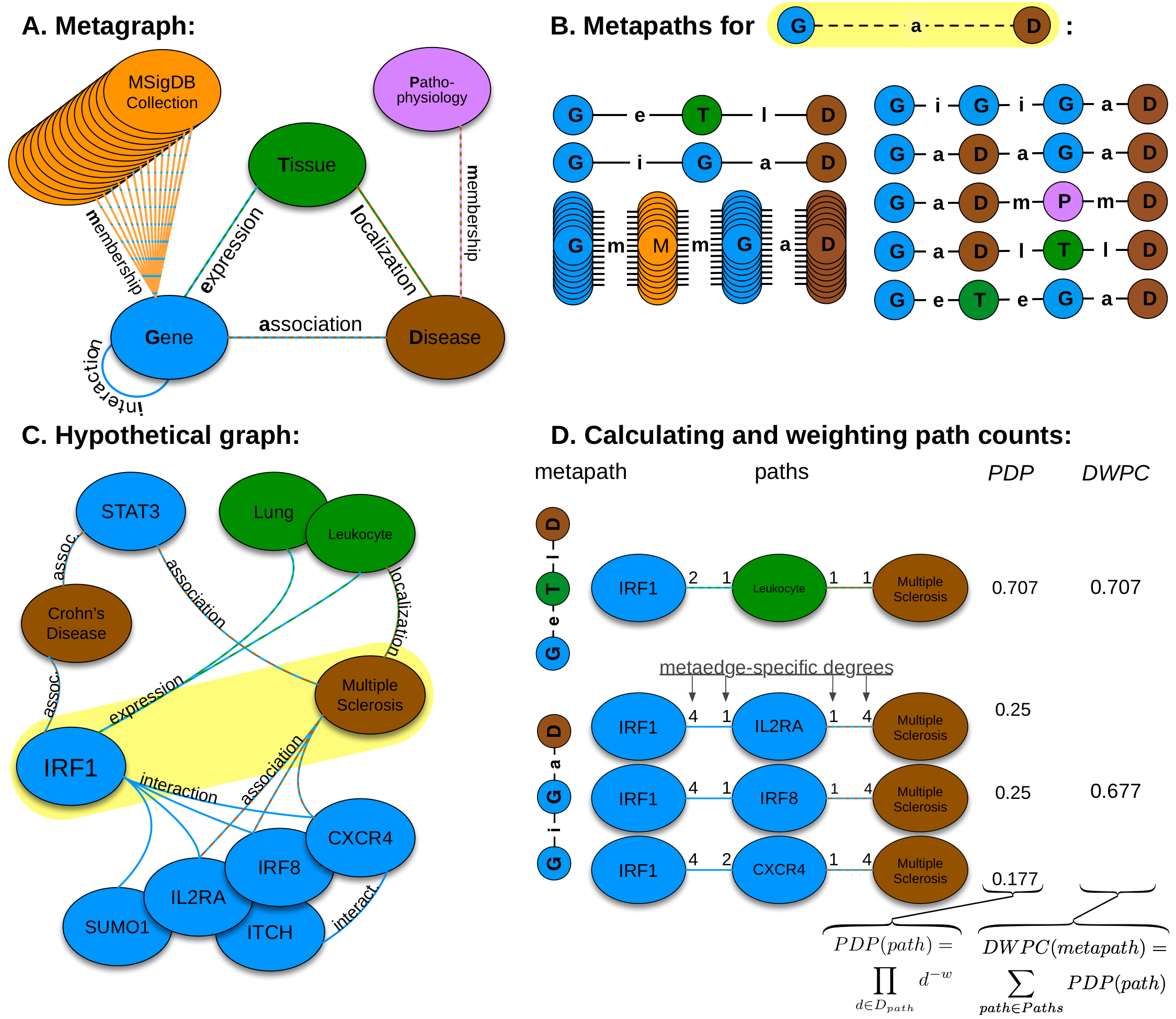# HNEP Heterogeneous Network Edge Prediction

## Heterogeneous Network Edge Prediction

The goal of HNEP is to produce biologically-meaningful predictions by integrating multiple high-throughput data sources. The approach computes features describing the network topology connecting two nodes. These features are used as input to a machine learning method which predicts the probability that an edge exists.

We adapted an alorithm originally developed for social network analysis called PathPredict [pdf, doi]. Our extensions to this method focused on scalability and performance.

## Methodology of Metapath-Based Edge Prediction

Here we show the process of calculating features that describe the network topology between two nodes. Features are used as predictors for subsequent modeling.1. We constructed the network according to a schema, called a metagraph, which is composed of metanodes (node types) and metaedges (edge types).
2. The network topology connecting a gene and disease node is measured along metapaths (types of paths). Starting on Gene and ending on Disease, all metapaths length three or less are computed by traversing the metagraph.
3. A hypothetical graph subset showing select nodes and edges surrounding IRF1 and multiple sclerosis. To characterize this relationship, features are computed that measure the prevalence of a specific metapath between IRF1 and multiple sclerosis.
4. Two features (for the GeTlD and GiGaD metapaths) are calculated to describe the relationship between IRF1 and multiple sclerosis. The metric underlying the features is degree-weighted path count (DWPC). First, for the specified metapath, all paths are extracted from the network. Next, each path receives a path-degree product measuring its specificity (calculated from node-degrees along the path, Dpath). This step requires a damping exponent (here w = 0.5), which adjusts how severely high-degree paths are downweighted. Finally, the path-degree products are summed to produce the DWPC.